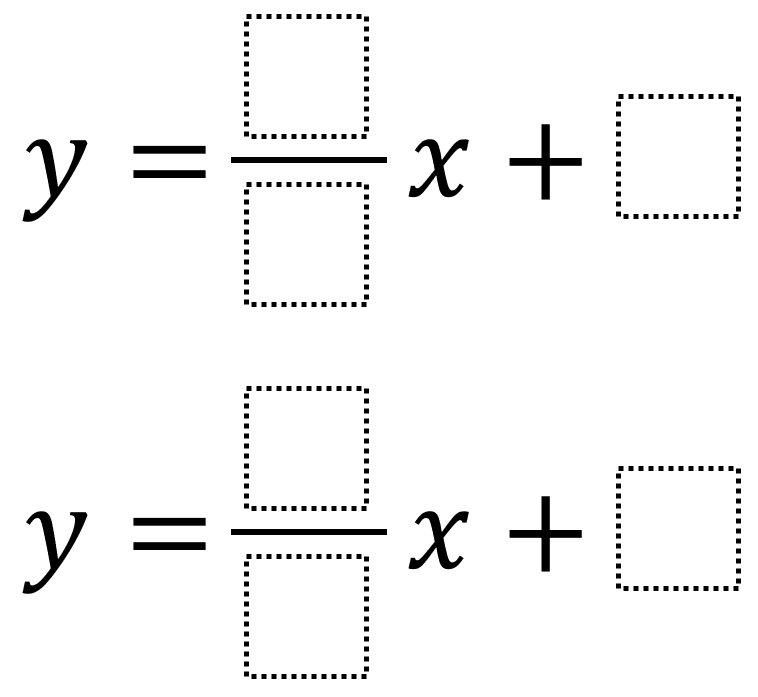# Systems of Equations 1

Directions: Using the integers -9 to 9 at most one time each, fill in the boxes to create a system of equations with a solution in Quadrant 2.### Hint

Where is Quadrant 2? How do the lines’ slopes affect the location of the solution? How do the lines’ y-intercepts affect the location of the solution?

There are many possible answers including y = (-1/2)x + 4 and y = (0/8)x + 5 which has a solution at (-2, 5).

Source: Robert Kaplinsky

## Exponential Powers

Directions: Using the digits 0 to 9 at most one time each, place a digit …

### One comment

1.